# Arrays The concept of arrays Using arrays Arrays

• Slides: 24Arrays The concept of arrays Using arrays Arrays as arguments Processing an arrays data Multidimensional arrays Sorting data in an array Searching with linear or binary search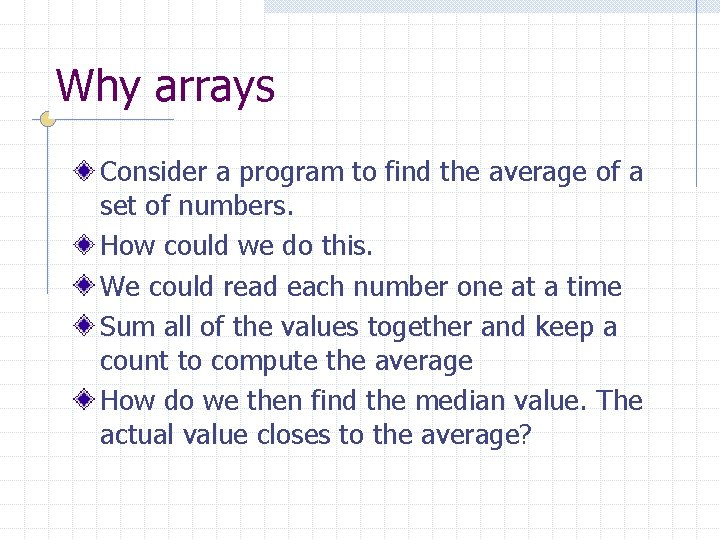Why arrays Consider a program to find the average of a set of numbers. How could we do this. We could read each number one at a time Sum all of the values together and keep a count to compute the average How do we then find the median value. The actual value closes to the average?Consider a list of 3 numbers. Number 1=5 Number 2=10 Number 3=7 Which is the median. How did we find that value? What would the code look like?The median sum = n 1+n 2+n 3 ave=sum/3 If (Math. abs(ave-n 1) < Math. abs(ave-n 2) && Math. abs(ave-n 1) < Math. abs(aven 3) ) median = n 1 Else …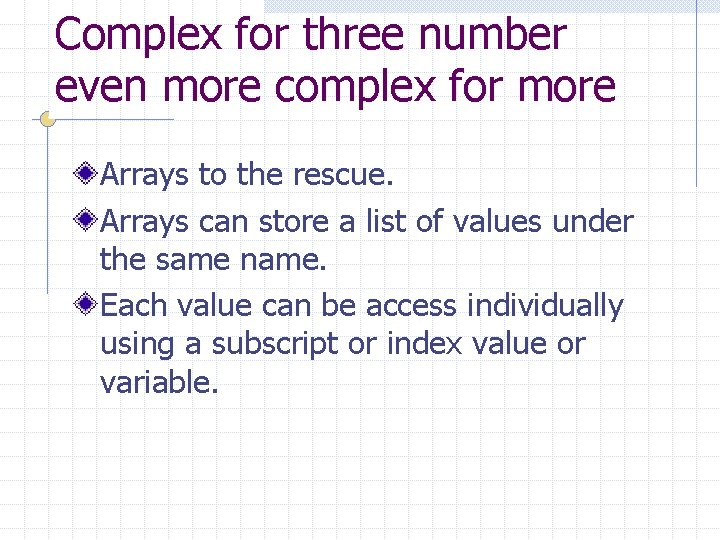Complex for three number even more complex for more Arrays to the rescue. Arrays can store a list of values under the same name. Each value can be access individually using a subscript or index value or variable.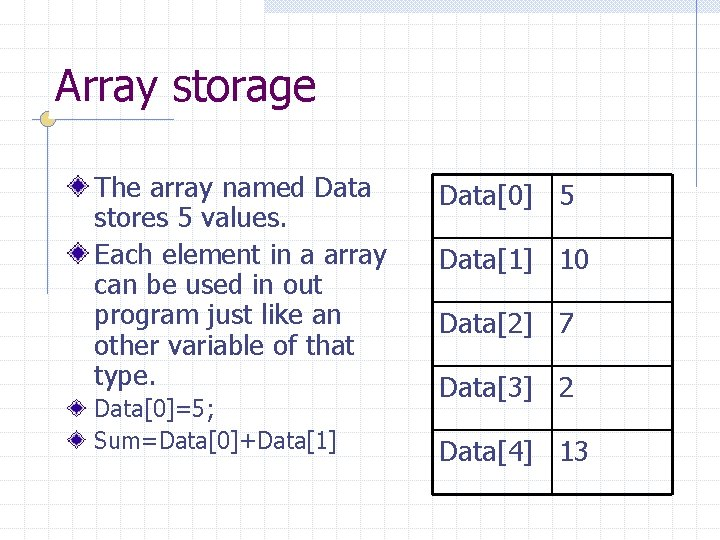Array storage The array named Data stores 5 values. Each element in a array can be used in out program just like an other variable of that type. Data=5; Sum=Data+Data Data 5 Data 10 Data 7 Data 2 Data 13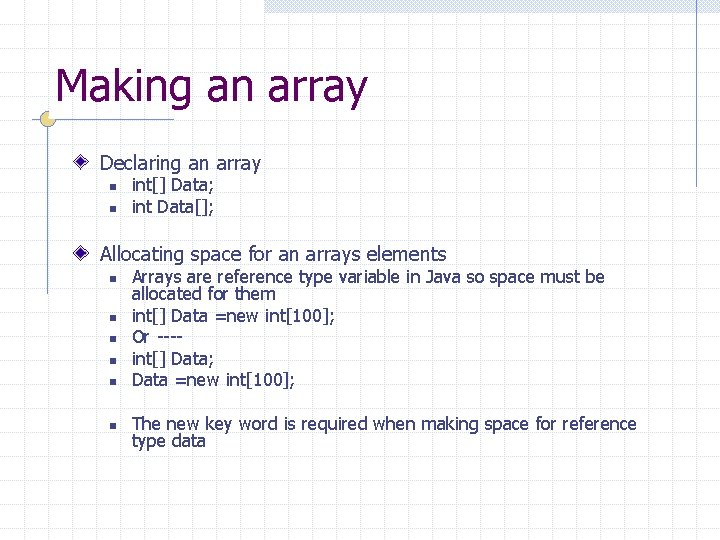Making an array Declaring an array n n int[] Data; int Data[]; Allocating space for an arrays elements n n n Arrays are reference type variable in Java so space must be allocated for them int[] Data =new int; Or ---int[] Data; Data =new int; The new key word is required when making space for reference type dataProperties of arrays Indexing begins at zero The. length property returns the number of elements in the array. So Data. length would be 100 It is an error to access a data element in a array that is not defined. This will result in a logic error that can be difficult to debug.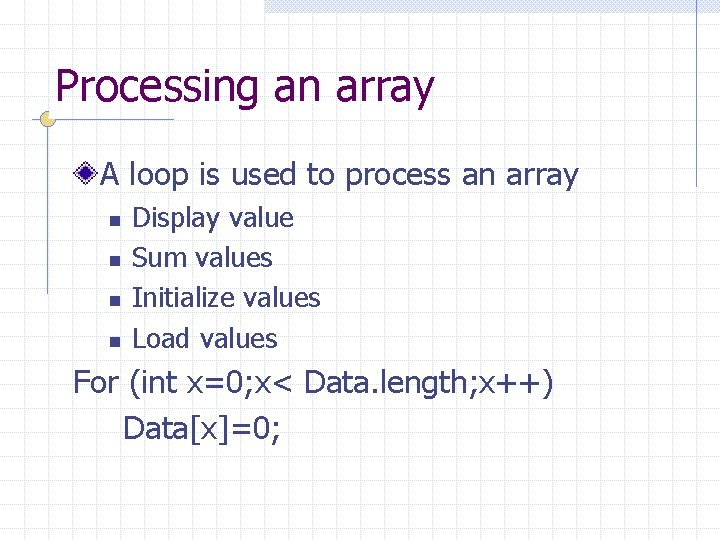Processing an array A loop is used to process an array n n Display value Sum values Initialize values Load values For (int x=0; x< Data. length; x++) Data[x]=0;Processing arrays When working with arrays it is common practice to create a variable to keep track of the actual valid data items in the array. Arrays may be declared at an arbitrarily large side the actual count of valid data elements in the array may be less. If this is the case then using the arrays length property is not valid. For (int x=0; x< Data_cnt; x++) System. out. println(Data[x]);Initialization lists String[] Month ={“”, ”Jan”, ”Feb”, …”Dec” }; System. out. println(Month); int[] Data={ 5, 10, 7, 12, 2 }; Can only be done when the array is declared or allocated So this can be done later in the program Data = new int[] { 5, 2, 5, 3, 1 };Lets make an example Write a program to collect grade scores from the user and store into an array. Write a loop to total the scores Write a loop to find the largest value Write a loop to count the number of time the largest value is entered.sum=0; // compute the total for(i=0; i<data_cnt; i++) sum=sum+data[i]; Sample JOption. Pane. show. Message. Dialog(null, "The sum is"+sum, "Results", JOption. Pane. PLAIN_MESSAGE); largest = 0; // find the largest value for(i=0; i<data_cnt; i++) { if (data[i] > largest) largest = data[i]; } JOption. Pane. show. Message. Dialog(null, "The largest is"+largest, "Results", JOption. Pane. PLAIN_MESSAGE); import javax. swing. *; public class grades { largest_cnt=0; // Count the number of times the largest value appears in the list for(i=0; i<data_cnt; i++) { if (data[i] == largest) largest_cnt ++; public static void main (String arg[]) { String snum; int num 1=0; int i, sum; int largest_cnt; } JOption. Pane. show. Message. Dialog(null, "The count of largest is"+largest_cnt, "Results", JOption. Pane. PLAIN_MESSAGE); int[] data = new int; int data_cnt=0; System. exit(0); // read in the numbers while (( num 1>=0 ) && (data_cnt<100)) { snum = JOption. Pane. show. Input. Dialog("Enter a Number? "); num 1=Integer. parse. Int(snum); data[data_cnt] = num 1; data_cnt++; } data_cnt--; } } // print the list for(i=0; i<data_cnt; i++) { System. out. print(" " + data[i] + " "); } sum=0; // compute the total for(i=0; i<data_cnt; i++) sum=sum+data[i]; JOption. Pane. show. Message. Dialog(null, "The sum is"+sum, "Results", JOption. Pane. PLAIN_MESSAGE);Using arrays in an assignment newlist = list This assignment would seem like it should copy the values from list to newlist. It does not. Remember that an array is a reference type data. So this actually causes newlist and list to reference that same data. list newlist List elements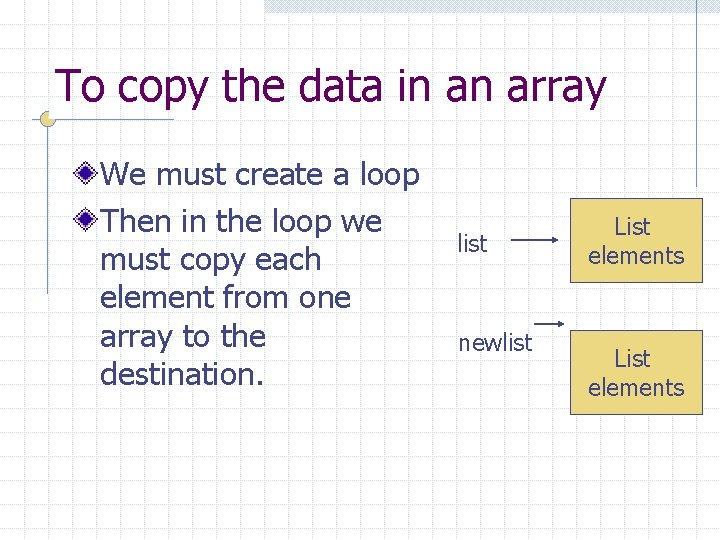To copy the data in an array We must create a loop Then in the loop we must copy each element from one array to the destination. list newlist List elements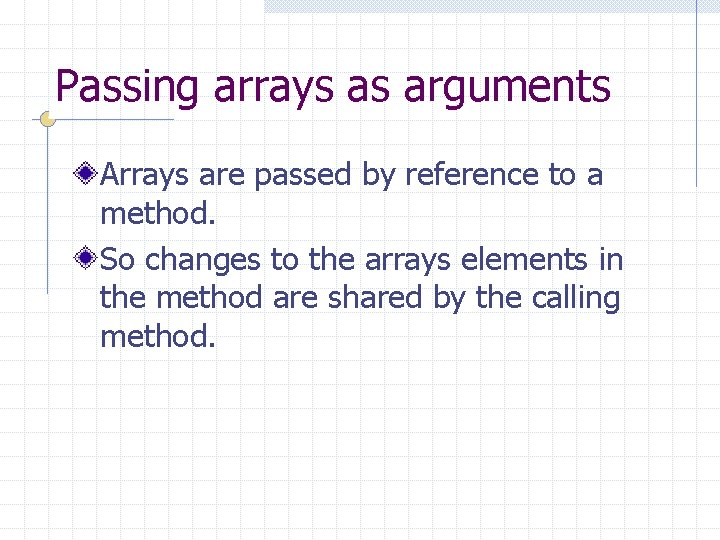Passing arrays as arguments Arrays are passed by reference to a method. So changes to the arrays elements in the method are shared by the calling method.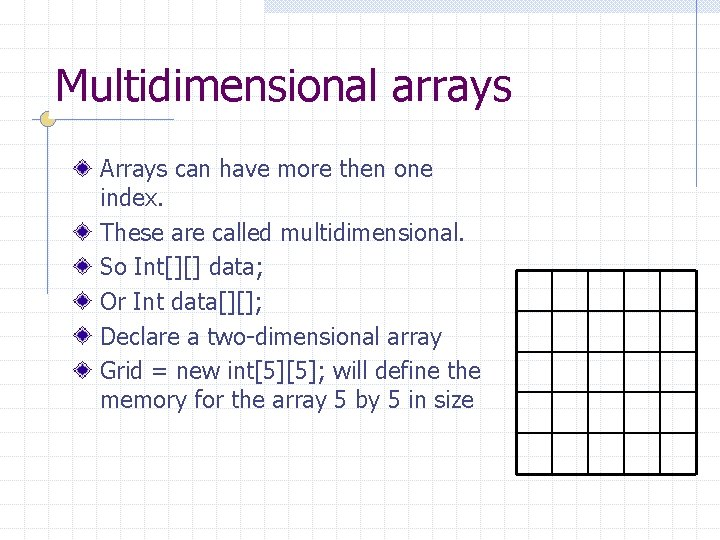Multidimensional arrays Arrays can have more then one index. These are called multidimensional. So Int[][] data; Or Int data[][]; Declare a two-dimensional array Grid = new int; will define the memory for the array 5 by 5 in size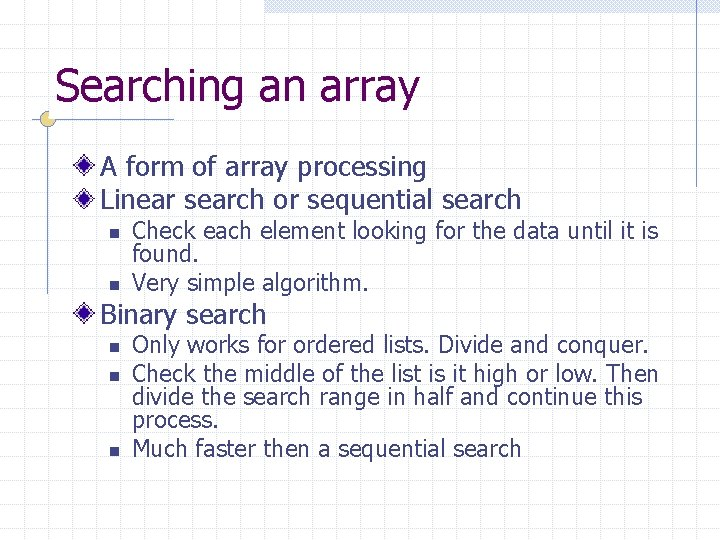Searching an array A form of array processing Linear search or sequential search n n Check each element looking for the data until it is found. Very simple algorithm. Binary search n n n Only works for ordered lists. Divide and conquer. Check the middle of the list is it high or low. Then divide the search range in half and continue this process. Much faster then a sequential search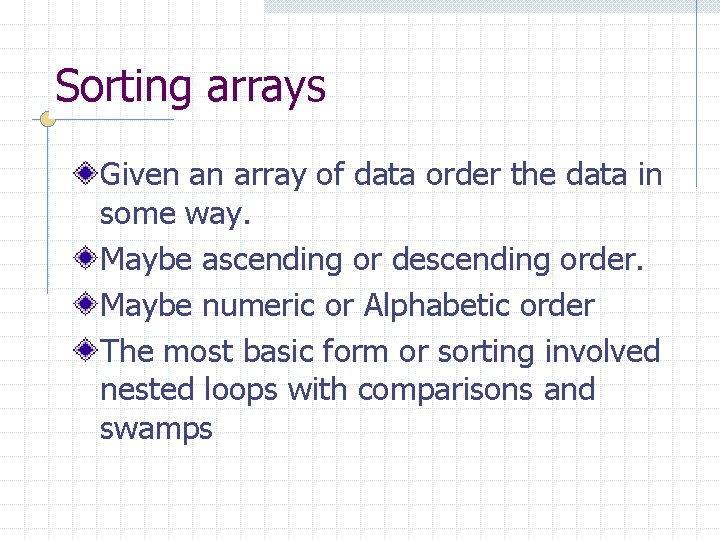Sorting arrays Given an array of data order the data in some way. Maybe ascending or descending order. Maybe numeric or Alphabetic order The most basic form or sorting involved nested loops with comparisons and swamps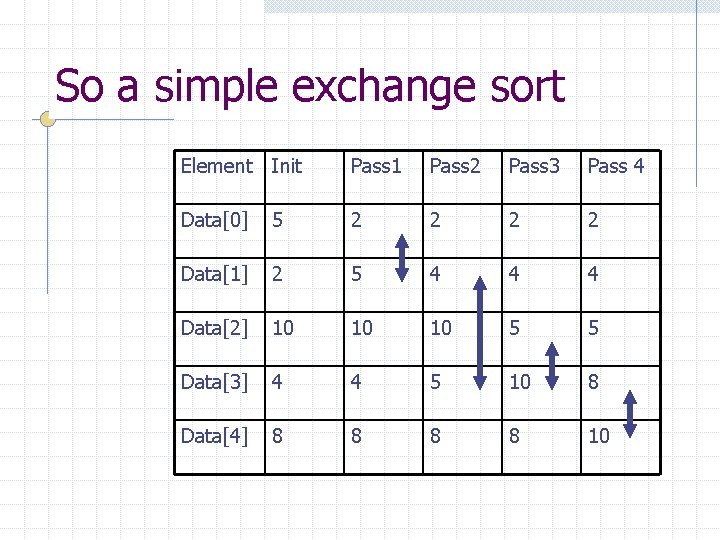So a simple exchange sort Element Init Pass 1 Pass 2 Pass 3 Pass 4 Data 5 2 2 Data 2 5 4 4 4 Data 10 10 10 5 5 Data 4 4 5 10 8 Data 8 8 10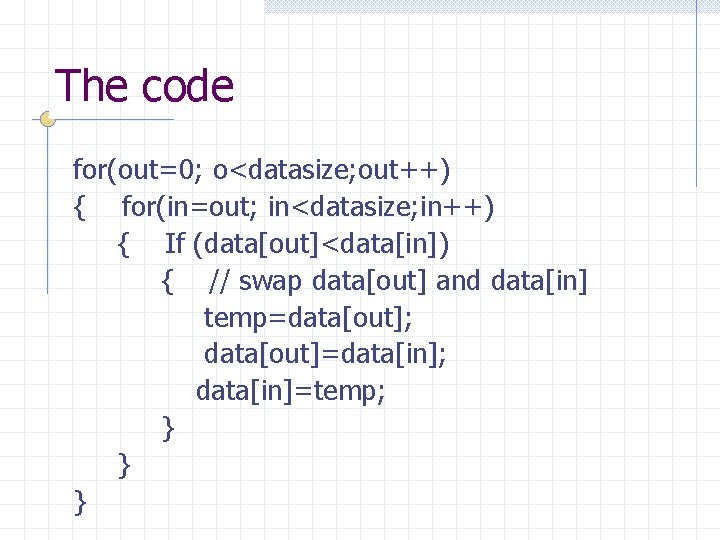The code for(out=0; o<datasize; out++) { for(in=out; in<datasize; in++) { If (data[out]<data[in]) { // swap data[out] and data[in] temp=data[out]; data[out]=data[in]; data[in]=temp; } } }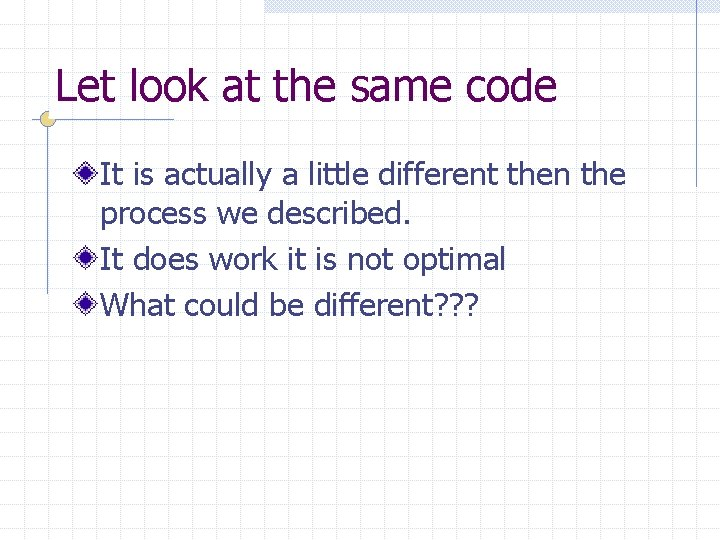Let look at the same code It is actually a little different then the process we described. It does work it is not optimal What could be different? ? ?An intuitive sort algorithm Try to find the lowest value in a list then swap it with the value in the first array slot. Then find the next lowest value in the list and try to swap it with the second array slot. Continue this process for each array slot up to the end of the list.Homework TBD